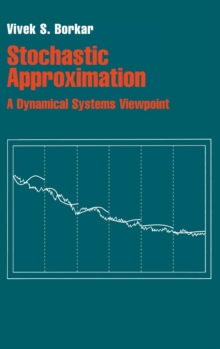Supporting your high street Find out how »
• My AccountStochastic Approximation : A Dynamical Systems Viewpoint Hardback

Description

This simple, compact toolkit for designing and analyzing stochastic approximation algorithms requires only basic literacy in probability and differential equations.

Yet these algorithms have powerful applications in control and communications engineering, artificial intelligence and economic modelling.

The dynamical systems viewpoint treats an algorithm as a noisy discretization of a limiting differential equation and argues that, under reasonable hypotheses, it tracks the asymptotic behaviour of the differential equation with probability one.

The differential equation, which can usually be obtained by inspection, is easier to analyze.

Novel topics include finite-time behaviour, multiple timescales and asynchronous implementation.

There is a useful taxonomy of applications, with concrete examples from engineering and economics.

Notably it covers variants of stochastic gradient-based optimization schemes, fixed-point solvers, which are commonplace in learning algorithms for approximate dynamic programming, and some models of collective behaviour.

Three appendices give background on differential equations and probability.

Information

• Format: Hardback
• Pages: 176 pages
• Publisher: Cambridge University Press
• Publication Date:
• Category: Probability & statistics
• ISBN: 9780521515924

£49.99

£42.85

on all orders

Pick up orders

from local bookshops